2022-10-1200

## 239.滑动窗口最大值

### 题目描述

``````输入：nums = [1,3,-1,-3,5,3,6,7], k = 3

---------------               -----
[1  3  -1] -3  5  3  6  7       3
1 [3  -1  -3] 5  3  6  7       3
1  3 [-1  -3  5] 3  6  7       5
1  3  -1 [-3  5  3] 6  7       5
1  3  -1  -3 [5  3  6] 7       6
1  3  -1  -3  5 [3  6  7]      7``````

``````输入：nums = , k = 1

• `1 <= nums.length <= 105`
• `-104 <= nums[i] <= 104`
• `1 <= k <= nums.length`

### 基本思路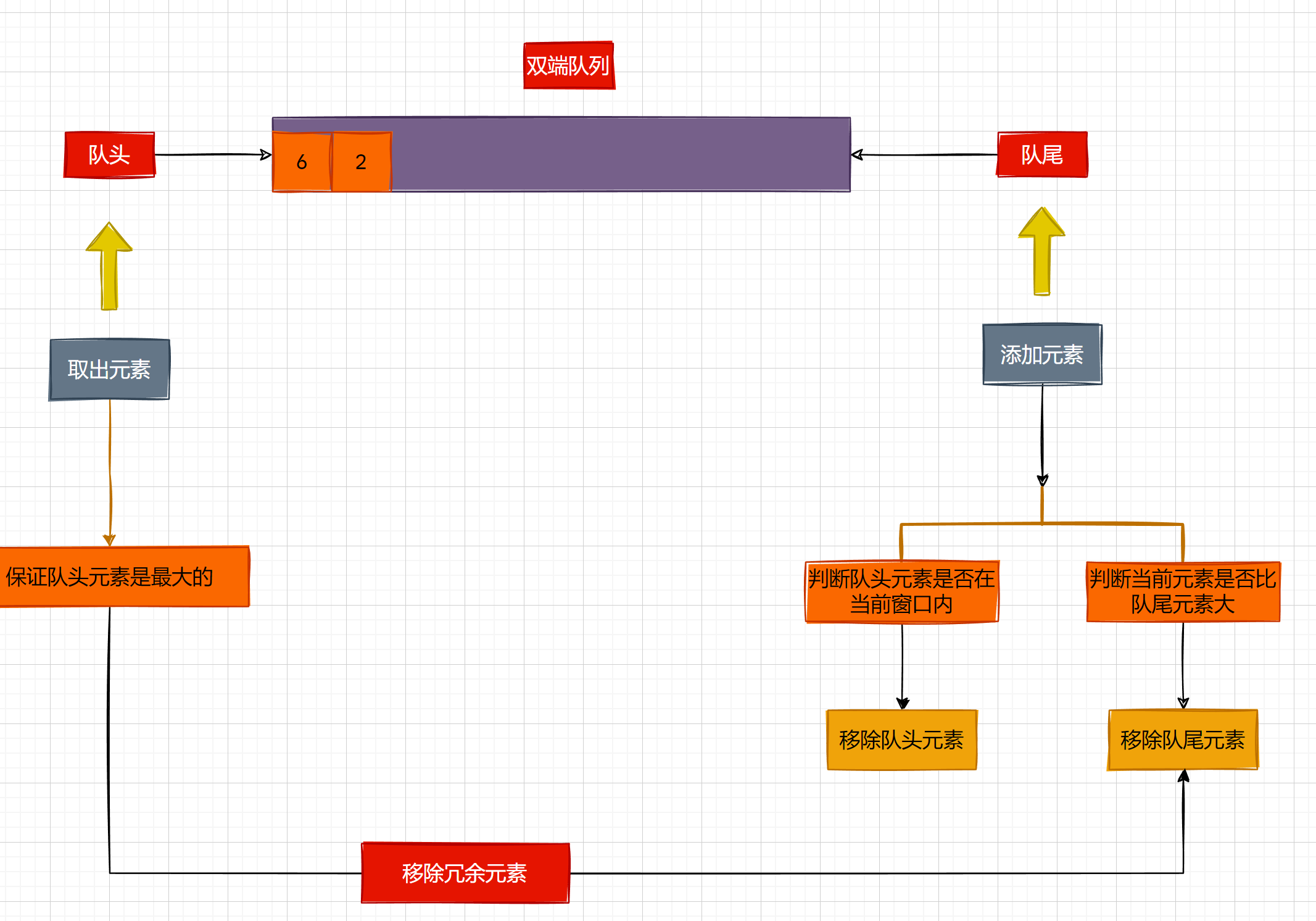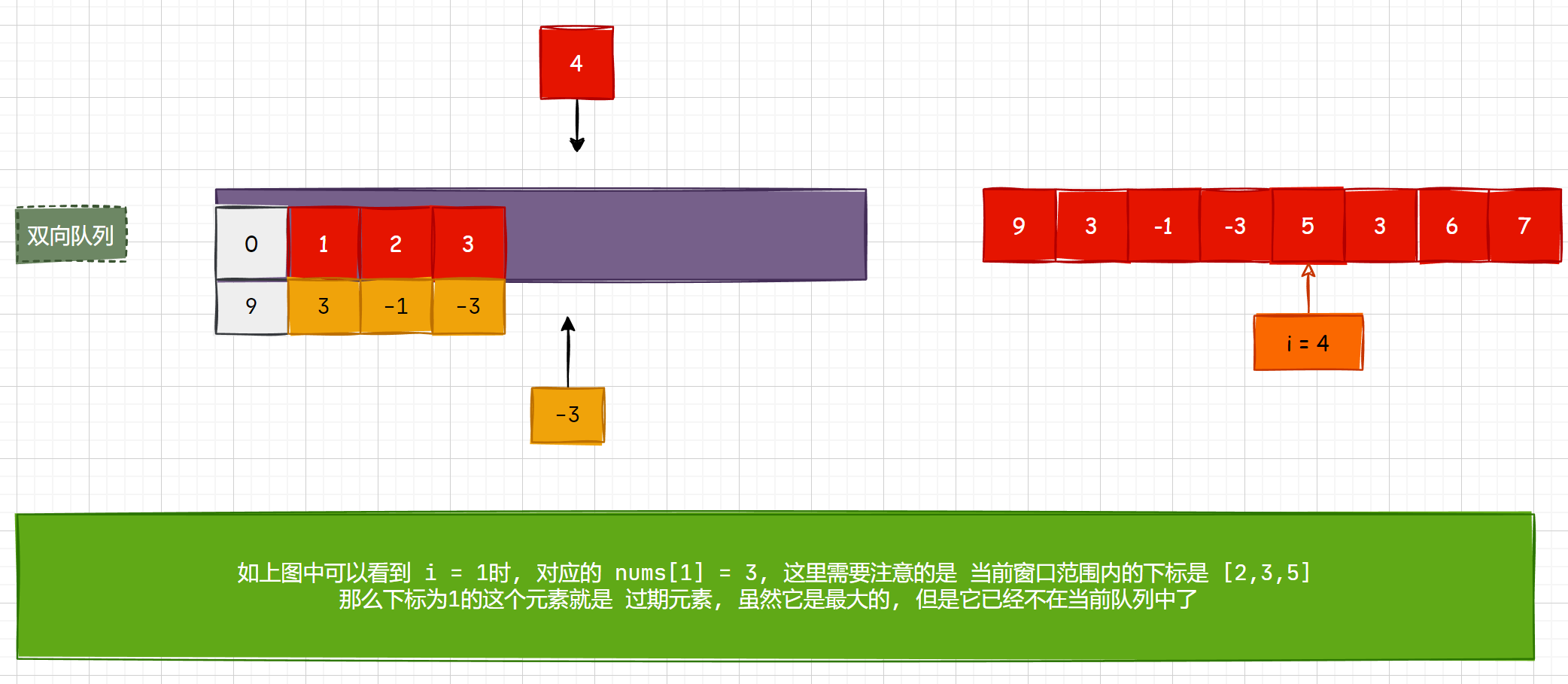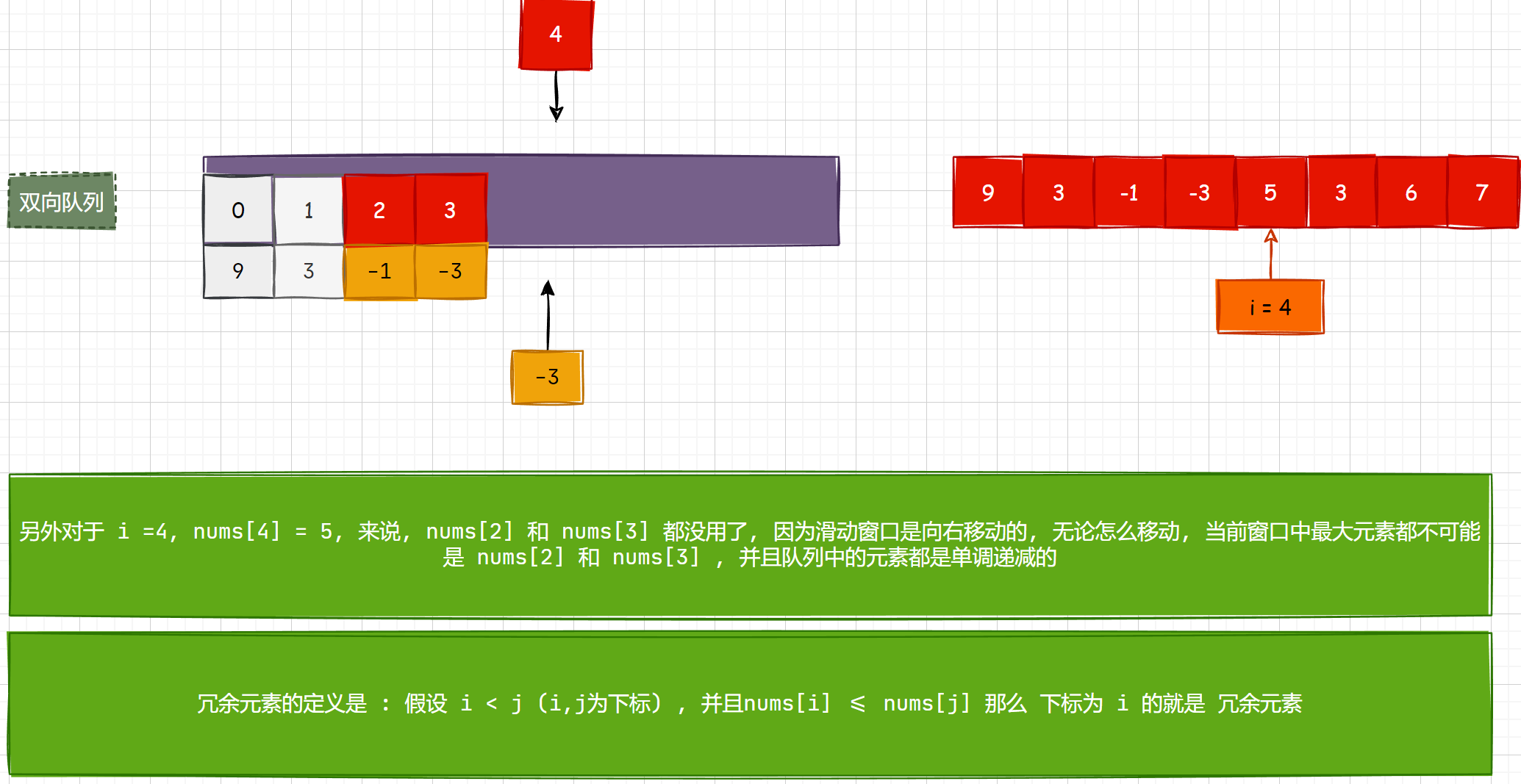### 思路图解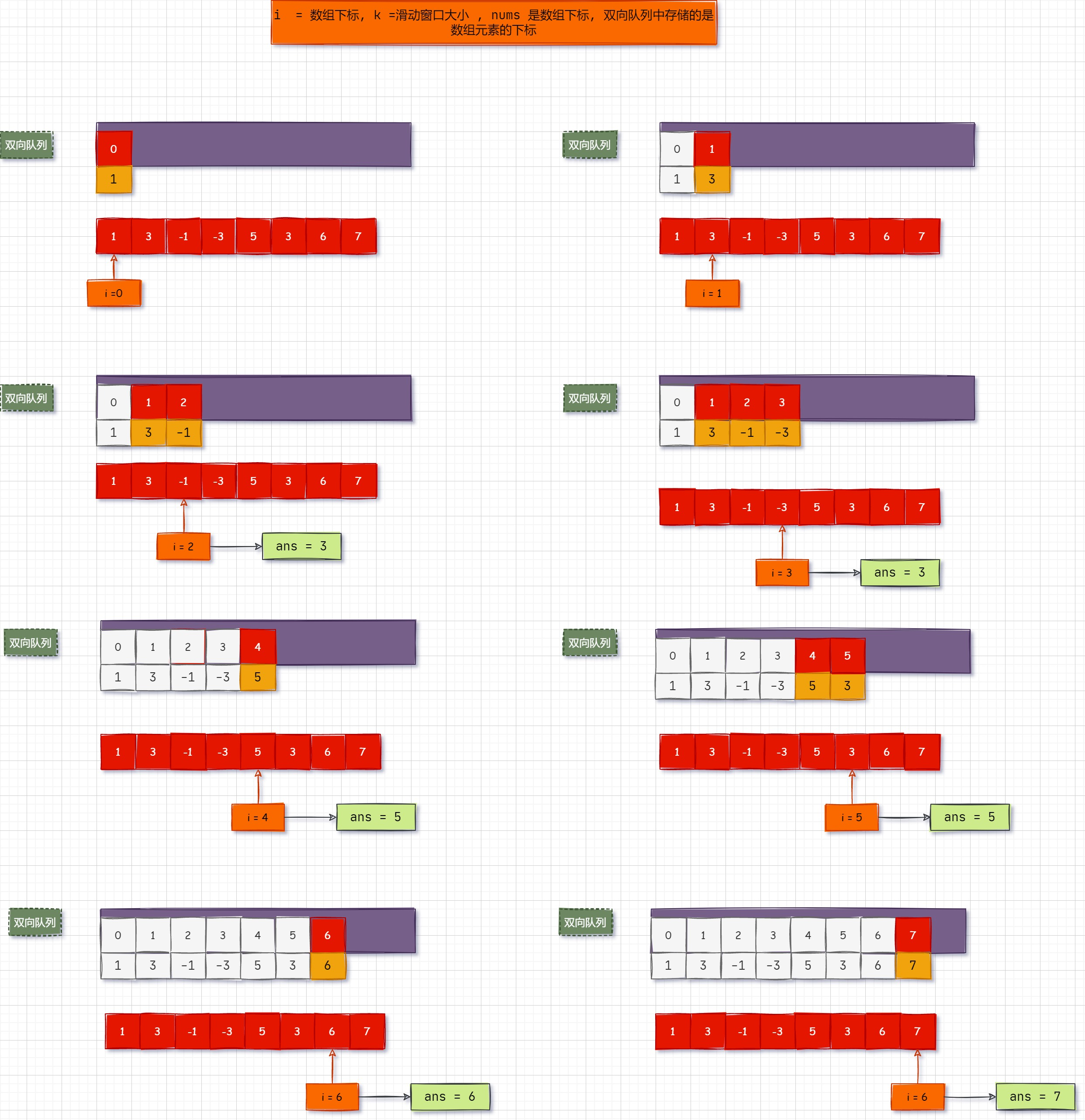• 白色的块是被移除的元素

• 遍历 nums 数组, 将元素放入数组中, 等到 i >= k -1 (第一个窗口尾部元素的时候) , 返回 队头元素
• 需要注意的是, 我们在把元素放入的时候, 只要后面的元素比前面的元素大, 就要把前面的元素移除掉, 因为我们要保证, 队头元素是当前窗口最大的元素
• 另外就是, 我们需要考虑队头过期的情况, 就是队头元素特别大, 后面的元素没有比它大的, 这样在后面的元素添加的时候它就不会被移除掉
• 但是当它过期, 也就是不在滑动窗口内的时候, 我们就要把它移除掉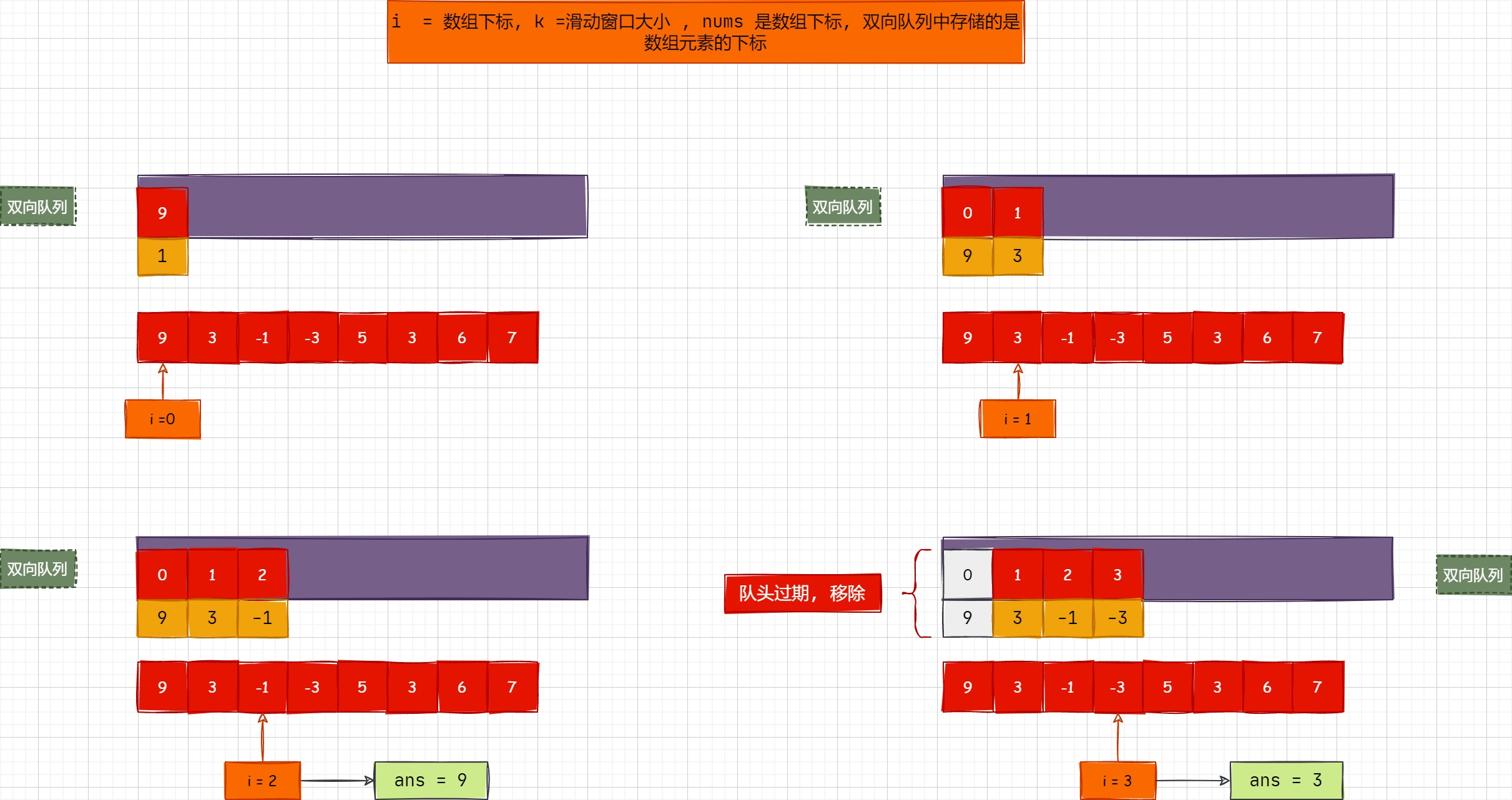``````				 // 队头过期 :
// 队头元素已经不在窗口内了
// i 是队尾元素, i - k 是最后一个元素
// 队头过期肯定是在第二个窗口才会出现过期的现象
while (!deque.isEmpty() && deque.getFirst() <= i - k) {
// 返回并删除队列头元素
deque.removeFirst();
}``````

#### 代码流程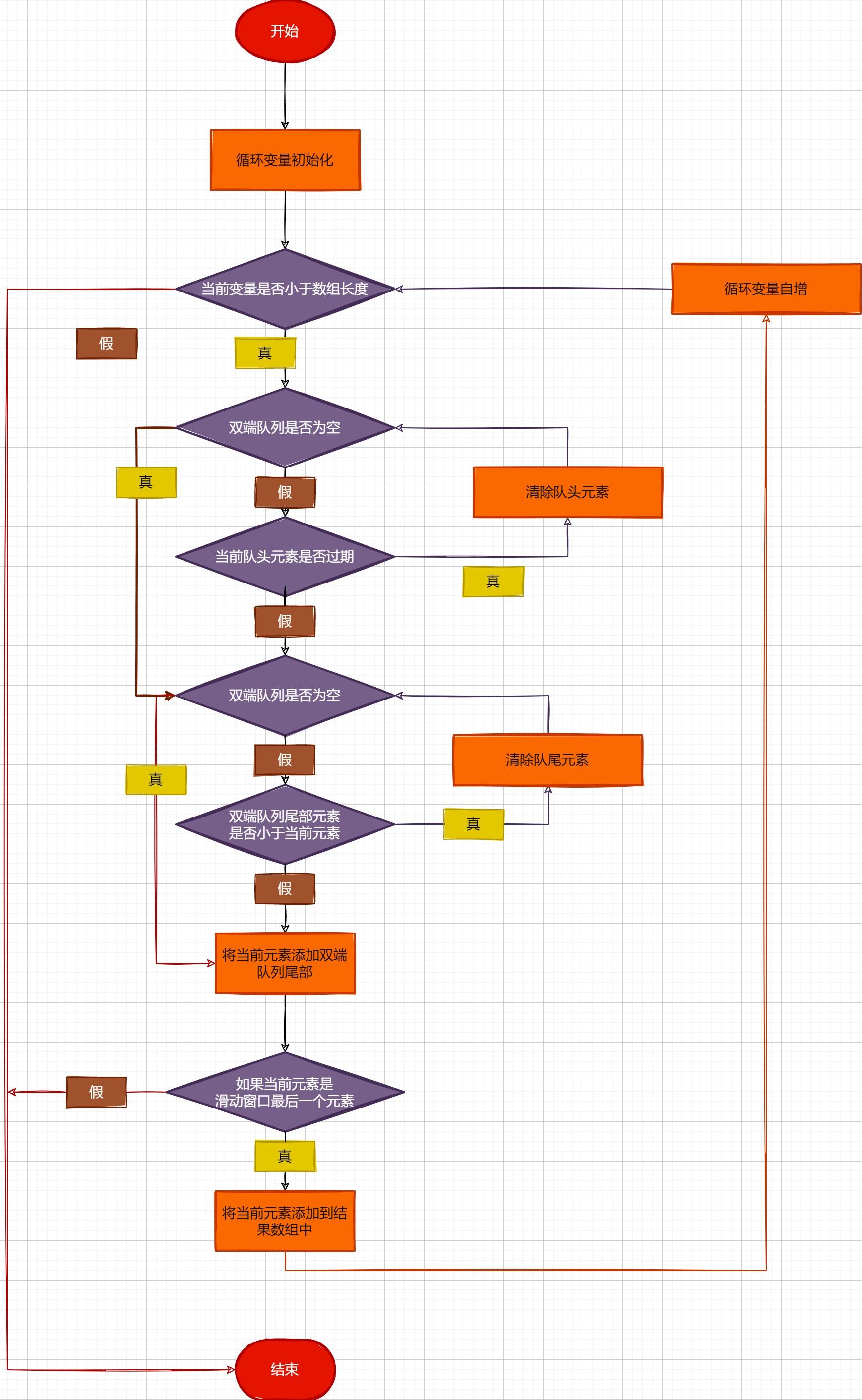### 代码实现

``````package leetcode.editor.cn;

import java.util.ArrayDeque;
import java.util.Deque;

/**
* 滑动窗口最大值
*
* @author
* @date 2022-10-04 18:19:29
*/
@SuppressWarnings("all")
public class SlidingWindowMaximum {
public static void main(String[] args) {
//测试代码
Solution solution = new SlidingWindowMaximum().new Solution();
int[] nums = new int[]{1, 3, -1, -3, 5, 3, 6, 7};
int k = 3;
solution.maxSlidingWindow(nums, k);
}

//leetcode submit region begin(Prohibit modification and deletion)
class Solution {
public int[] maxSlidingWindow(int[] nums, int k) {

int[] ans = new int[nums.length - k + 1];
int index = 0;
// 双端队列, 存储下标
Deque<Integer> deque = new ArrayDeque<>();
for (int i = 0; i < nums.length; i++) {
// 队头过期 :
// 队头元素已经不在窗口内了
while (!deque.isEmpty() && deque.getFirst() <= i - k) {
// 返回并删除队列头元素
deque.removeFirst();
}
// 如果nums[i] > nums[deque.getLast()]
// 说明队列中 前面的元素就都没用了, 因为 nums[i] 要比前面的元素都大
// 我们就要移除前面的元素, 要保证队头元素是最大的!
while (!deque.isEmpty() && nums[deque.getLast()] <= nums[i]) {
// 移除队尾元素
deque.removeLast();
}
// 取队头更新元素
if (i >= k - 1) {
ans[index] = nums[deque.getFirst()];
index++;
}
}
return ans;
}
}
//leetcode submit region end(Prohibit modification and deletion)
}
``````